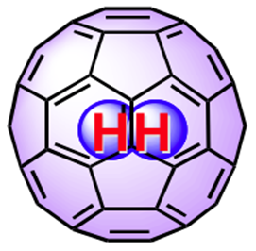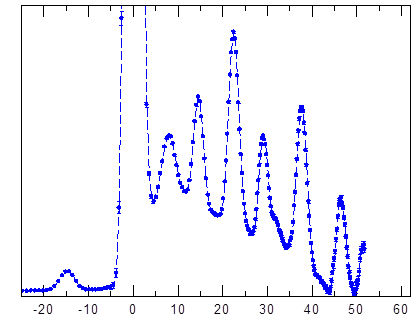# H2@C60The union of the simplest molecule (H2) and the most symmetric molecule (C60) known to men have enabled the observation of quantum mechanics at a molecular level. The truncated icosahedral symmetry of the C60 cage enable the guest molecule to behave like a three-dimensional quantum harmonic oscillator.

Due to symmetry requirements, para-H2 can only be in states where its rotational angular momentum quantum number, j, is even (0, 2, 4 ...), while ortho-H2 can only have odd j (1, 3, 5 ...). Inter-conversion between the two species is slow in the absence of catalyst as most typical intra-molecular interactions that causes spin flip are small and the energy difference between the rotational energy levels is large because interaction must flip space and spin states simultaneously. 

The dynamics of the hydrogen can be described in terms of 5 quantum numbers (ν, j, n, l, λ, m). The quantum number ν =0, 1… represents vibration, while j is as above, represents the rotation of the hydrogen molecule. The integer n is the principle quantum number that describes the displacement of the centre of mass of the hydrogen molecule from the centre of the cage. The quantum number l on the other hand, expresses the hydrogen molecule's translational angular momentum. This integer can only assume a positive value and is defined as l = n, n-2… until 1 or 0 depending if n is odd or even. λ is the quantum number that describes the vector sum of translation and rotational angular momentum,$\Lambda = L + J$, where$|L|=\hbar \sqrt{l(l+1)}$ and$|J|=\hbar \sqrt{j(j+1)}$.  The quantum number m is the projection of the total angular momentum along any quantization axis and it can take integer values from -λ to λ . However, in isotropic systems such as H2@C60, m is not a good quantum number due to the translational-rotational (TR) coupling. λ is the better quantum number as it is used to clarify the TR coupled states.

The energy of the H2 molecules can be written as:$E(\nu, j ,n, \lambda) = (v +\frac{1}{2}) \omega_\nu) + (n +\frac{3}{2}) \omega_n) + B_\nu j(j+1) + c(l, j, \lambda) V_{t-r}$ (Equation 1)

where$\hbar$ is the reduced Planck constant,$\omega_\nu$ is the vibrational quantum of energy,$\omega_n$ is the translational quantum of energy.$B_\nu = B_e - \alpha_e (\nu+\frac{1}{2})$ is the rotational constant with$\alpha_e$ representing the vibration-rotation (VR) correction. The last term represents the TR coupling that is responsible for the sub-level splitting, where$V_{t-r}$ is the TR coupling and$c(l, j, \lambda)$ is the coefficient. The results from the above equation can be plotted in an energy level diagram (Figure 1). The degeneracy of the energy levels in Equation 1 is expressed in terms of$g=2\lambda+1$.Figure 1. The inelastic neutron scattering (INS) spectrum of the H2@C60 at 1.5K.Figure 2. Energy levels of H2@C60 as observed from inelastic neutron scattering (INS) spectroscopy (see Figure 1). The numbers next to each energy level represents their respective quantum numbers, J, n and λ.

References:
1. A. J. Horsewill, K. S. Panesar, S. Rols, J. Ollivier, M. R. Johnson, M. Carravetta, S. Mamone, M. H. Levitt,Y. Murata, K. Komatsu, J. Y.-C. Chen, J. A. Johnson, X. Lei, and N. J. Turro (2012) Phys. Rev. B 85, 205440.
2. M. Xu, F. Sebastianelli, Z. Bacic, R. Lawler, and N. J. Turro. Quantum dynamics of coupled translational and rotational motions of H2 inside C60 (2008) J. Chem. Phys, 128:011101.